# Magic Cups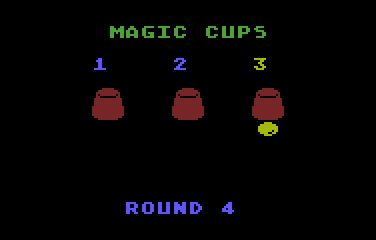This is a little game for the 2018 NOMAM's BASIC 10-liners Contest. This program fits in the PUR-120 category, and it was written using TurboBASIC XL 1.5 for the 8-bits ATARI XL/XE. Development started on 2018-02-09, and it took 1+1 days. The final version's date is 2018-02-10.

UPDATE: It obtained the 3rd place of 17 entries in the category.

## Description

Do not stop looking at where the little ball is. Whoops! Can you find out where it is now?

## Instructions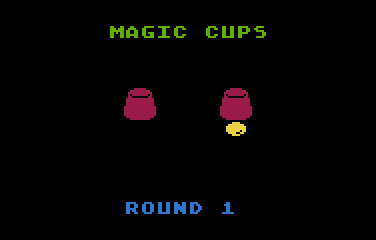The ball is covered by a cup.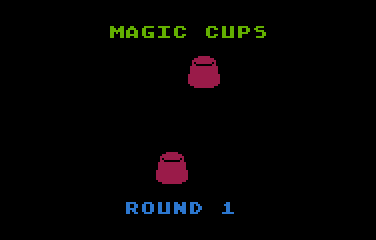The cups are mixed some times. Follow your cup with your eyes.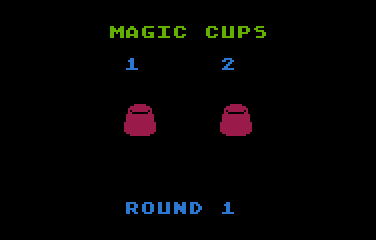Use the keyboard numbers to choose the cup where you think the ball is.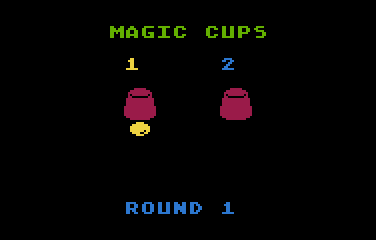The cups are raised to check for your selection.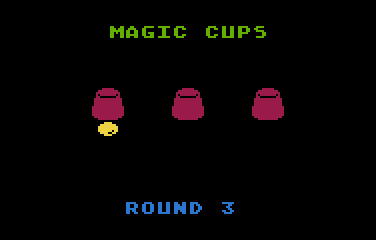If you were right, the ball is covered again, the cups are mixed faster, and from time to time, more cups are added.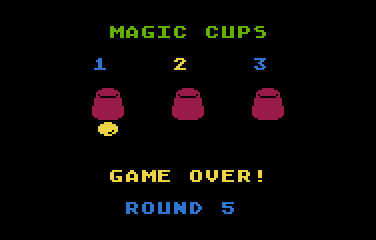The game is over when you lose the ball.

## Development of the game

I was thinking in a simple animation that could be performed in Atari BASIC for the PUR-80 category, and the idea of the sliding cups came into my mind. But using large objects is not something that could be done with single instructions and DPOKE was not available in that flavor of BASIC.

Anyway, I wanted to see how it performed a single swap animation using TurboBasic XL and then evaluate alternatives for Atari BASIC. After a couple of hours, I had a animation working in TurboBasic XL, but the size of the routine was larger than the expected for a PUR-80 category, so I continued the development for PUR-120 category, where TurboBasic XL is allowed.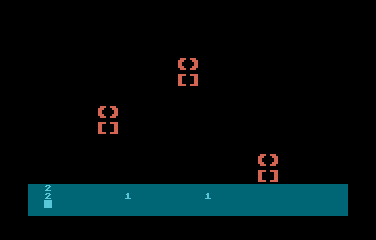Mixing prototype

The mixing routine worked so well, that any number of cups could be used. It was only required to identify the position of the first cup in the row and the distance between them to span smoothly in the screen. That gave me the idea of the game concept: few cups at the beginning and many in higher rounds. Also the number of swaps and the speed of them should be increased in the following rounds.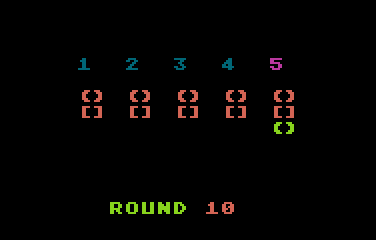Many cups

The next step was to add some graphics, so I designed very simple sprites. The game looked very different and that required the suitable sound effects, so I tried some variations and picked the best ones.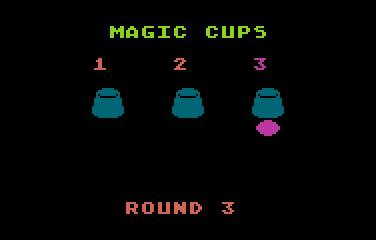First version of sprites

To complete the game, I selected 2 color palettes, one for PAL and one for NTSC. I also added the title and game over message, and adjust the sprites a little to add a better 3D effect.

A trivia of this game is that no IF-ENDIF conditional structures were used. The flow control is managed by a REPEAT-UNTIL and some FOR-NEXT loops, and other conditional behaviors are managed in expresions.

Get the MAGICCUP.ATR file and set it as drive 1 in a real Atari (or emulator). Turn on the computer and the game should start after loading.

## The code

The abbreviated BASIC code is the following: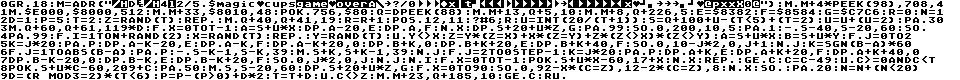The full and expanded BASIC listing is:

 ```graphics 18 ``` Sets a 20x12 text screen with 4 colours for the texts and another for the background. ```m=adr("{binary data}") ``` Binary data that contains: 1-4 (4 bytes): the NTSC color palette 5-8 (4 bytes): the PAL color palette 9-13 (5 bytes): "round" message 14-23 (10 bytes): game title 24-33 (10 bytes): game over message 34-81 (48 bytes): bitmap of the cups (4 fonts) and the ball (2 fonts) ```move m+4*peek(98),708,4 ``` Sets the color palette based on current video system (NTSC or PAL). The background remains in black for boths systems. ```move \$E000,\$8000,512 move m+33,\$8010,48 poke 756,\$80 ``` Copies the charset from ROM to RAM, modifies 6 fonts for the cups and the ball, and enables the new charset. ```q=dpeek(88) move m+13,q+5,10 move m+8,q+226,5 ``` Prints the fixed messages in screen. Q is the screen memory address. ```e=\$8382 f=\$8584 g=\$C7C6 ``` Assigns the fonts to be used for objects: E and F are the top and bottom half of the cup, and G is the ball. ```r=0 n=1 d=1 p=5 t=2 ``` Sets up the initial parameters for the first round. R is the round number, N is the number of swaps in a round, D is a flag that says when to add a cup in a round, P is the length of the pause during the animation, and T is the number of cups. ```z=rand(t) ``` Assigns a cup that holds the ball. ```repeat ``` Game loop: ``` move q+40,q+41,19 ``` Cleans the screen area where the cup's numbers appear. ``` r=r+1 position 12,11 ? #6;r ``` Updates the round number and prints that in the screen. ``` u=int(20/(t+1)) ``` Computes the distance between cups. ``` s=q+100+u-(t<5)+(t=2) ``` Computes the position of the first cup. ``` u=u+(u=2) ``` Adjusts the distance for the row with more cups. ``` pause 30 ``` Just a small delay. ``` move q+60,q+61,119*d ``` Cleans the playfield if the number of cups has changed. ``` for x = 0 to t-1 a=s+u*x dpoke a-20,e dpoke a ,f next x ``` Draws the row of cups. ``` dpoke s+20+u*z,g ``` Draws the ball in its current position. ``` pause 99 ``` Let the player see for a moment where the ball is. ``` sound 0,200,10,5 pause 1 -move s-40,s-20,60 sound ``` Moves down the cups, hiding the ball. ``` pause 99 ``` Ready to mix the cups? ``` for i=1 to n+rand(2) ``` Loop to swap cups. The number of swaps is not always the required... sometimes an extra swap is done. ``` x=rand(t) repeat y=rand(t) until y<>x ``` Chooses 2 cups randomly. ``` z=y*(z=x)+x*(z=y)+z*(z<>x)*(z<>y) ``` If any of the cups had the ball, swap it too. ``` a=s+u*x b=s+u*y ``` Sets the position in screen of each of the selected cups. ``` for j=0 to 2 k=j*20 pause p dpoke a-k-20,e dpoke a-k ,f dpoke a-k+20,0 dpoke b+k ,0 dpoke b+k+20,e dpoke b+k+40,f sound 0,10-j*2,0,j+1 next j ``` The first selected cup is moved upwards and the other is moved downwards. ``` k=sgn(b-a)*60 for j=1 to abs(b-a) pause p -move s-k-1,s-k,39 move s+k,s+k-1,39 next j ``` Moves the cups horizontally. It uses K to help which row sould be moved to the left and which one to the right. The number of moves is based on the distance between the cups. ``` for j=2 to 0 step -1 k=j*20 pause p dpoke a+k ,e dpoke a+k+20,f dpoke a+k+40,0 dpoke b-k-20,0 dpoke b-k ,e dpoke b-k+20,f sound 0,j*2,0,j next j ``` The second cup arrived to the position of the first one, so the cup in that position must be moved upwards again to line it up in the row. The other must be moved downwards at the same time. ``` next i ``` End of the swap loop. ``` for x = 0 to t-1 poke s+u*x-60,17+x next x ``` Draws a number over each cup. ``` repeat get c c=c-49 until c>=0 and c0)+d*2 t=t+d ``` Adjust parameters for the next round. Max number of swaps is 20 and the max number of cups is 6. The delay in the animation decreases in each round, but it is increased a bit when a new cup is added. ```until c<>z ``` Go to the next round only if the player hit. ```move m+23,q+185,10 ``` Displays the game over message. ```get c run ``` Waits for a new game.